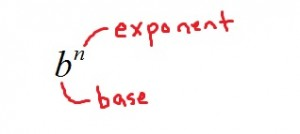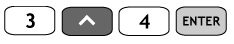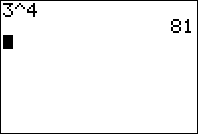﻿ Understanding Exponents - MathBootCamps

# Understanding Exponents

When working with exponents, there are two new words that come up: exponent and base. As an example, in $$x^2$$, 2 is the exponent while $$x$$is the base and in $$4^{12}$$, 12 is the exponent while 4 is the base.## Whole number exponents are about multiplication

As long as the exponent is a positive whole number, you can think of it as telling you how many times you should multiply the base by itself.

$$2^3=2\cdot2\cdot2=8$$
$$x^3=x\cdot x\cdot x$$
$$5^2=5\cdot5=25$$

As you can see,  $$4^{12}$$ means “4 multiplied by itself 12 times” and that is a really big number. Many values that you calculate from exponents will be quite large.

There are a couple of things to note:

• Anything with an exponent of zero is defined to be 1. So, $$4^{0}=1$$, $$100^{0}=1$$.
• Ok right above, when I said anything, I meant “almost anything”. $$0^0$$ is an indeterminate form. For everything else however, the rule above holds.
• An exponent of 1 is the same as just writing the number by itself: $$4^{1}=4$$.

## Negative Exponents

Negative exponents are treated a bit differently. By definition, if we have a positive number $$n$$ and a nonzero $$b$$, then the following rule holds:

$$b^{-n} = \dfrac{1}{b^n}$$

Using this rule, if you see a value with a negative exponent, then it can be rewritten as 1 “over” that term with a positive exponent. Here are a few examples of how this works.

$$3^{-2} = \dfrac{1}{3^2} = \dfrac{1}{9}$$

$$10^{-1} = \dfrac{1}{10^1} = \dfrac{1}{10}$$

$$(-2)^{-4} = \dfrac{1}{(-2)^4} = \dfrac{1}{16}$$

As you can see, it is simply about following the formula when dealing with negative exponents. After this, you can simply calculate the value using the rules above.

## Fractions as exponents

In algebra and more advanced math, it is very common to see an exponent of one-half or some other fraction. This is one way to represent terms involving roots, or radical terms. By definition (if n is nonzero):

$$b^{\frac{m}{n}}= \sqrt[n]{b^m}$$

Working with these types of terms (radical terms) is a more advanced topic and will be the subject of a different article, but it is important that you be able to rewrite such terms using the definition as below.

$$3^{\frac{1}{2}}=\sqrt{3^1}=\sqrt{3}$$

$$4^{\frac{2}{3}}=\sqrt{4^2} = \sqrt{16}$$

As you study terms involving exponents, you will find that you need to combine rules quite often. For example, the rule above can be combined with the rule for negative exponents to simplify even more complex expressions.

## Using a Calculator

Finding the value of terms with exponents on scientific or graphing calculators is quite easy. The usual key you will need will use a carat symbol ^ to represent that the next number is an exponent. So, $$3^4$$ can be represented as 3^4 in most calculators. On the TI83 or TI84:You can also always use the carat symbol on google (just type it into google! they now do automatic calculations for you) or wolfram alpha.